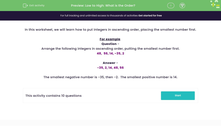# Practise Ordering Numbers

In this worksheet, students will arrange positive and negative integers in increasing order of size.Key stage:  KS 2

Curriculum topic:   Number: Number and Place Value

Curriculum subtopic:   Solve Number Problems to 1 000 000

Popular topics:   Ordering Numbers worksheets

Difficulty level:#### Worksheet Overview

In this worksheet, we will learn how to put integers in ascending order, placing the smallest number first.

Don't be put off by the word integer - it simply means a whole number, not a fraction or a decimal.

For example:

Arrange the following integers in ascending order, putting the smallest number first.

48,  56, 14, -35, -2

-35, -2, 14, 48, 56

The smallest negative number is -35, then -2.  The smallest positive number is 14.

Let's try another one:

Arrange the following integers in ascending order:

12, 0, -3, -15, 5

-15, -3, 0, 5, 12

Let's move on to some questions now.

### What is EdPlace?

We're your National Curriculum aligned online education content provider helping each child succeed in English, maths and science from year 1 to GCSE. With an EdPlace account you’ll be able to track and measure progress, helping each child achieve their best. We build confidence and attainment by personalising each child’s learning at a level that suits them.

Get started